# JEE-MathematicalToolsinPhysics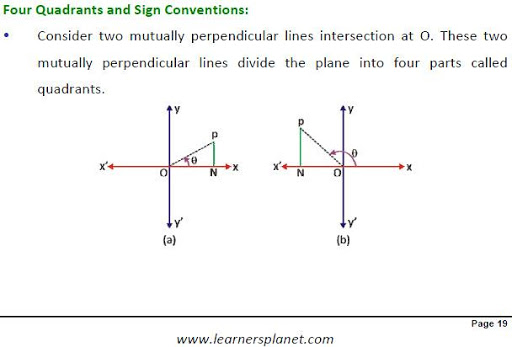This book discusses common mathematical formulae used in physics, Sequences, elementary trigonometry, Polynomial, Linear and Quadratic Equations, Elementary Calculus etc.

Magazine Description:

Discusses elementary trigonometry, Basic Trigonometric Ratios, Relations between basic ratios Trigonometric Formulae, Inverse Trigonometric Functions Solved Example, Polynomial, Linear, and Quadratic Equations, Quadratic Equations: Inequalities Binomial Expression and Theorem, Solved Example ,Coordinate Geometry Graphs for Common Functions. Elementary Calculus, Geometrical interpretation, Quotient Rule ,Chain Rule ,Derivative of some important Functions, Maxima and Minima, Application in Physics, Solved Example, Integration Definite Integral Application in Physics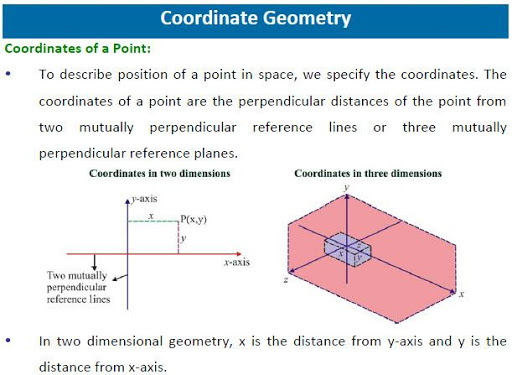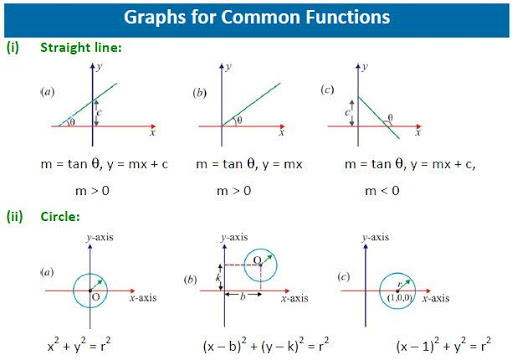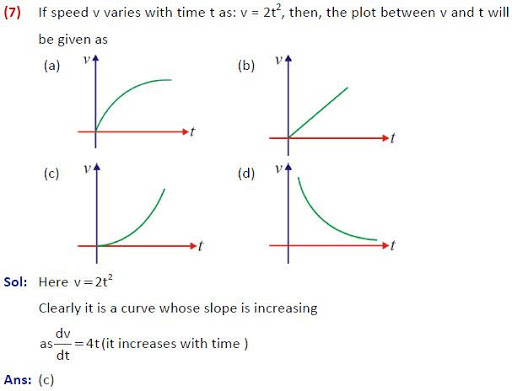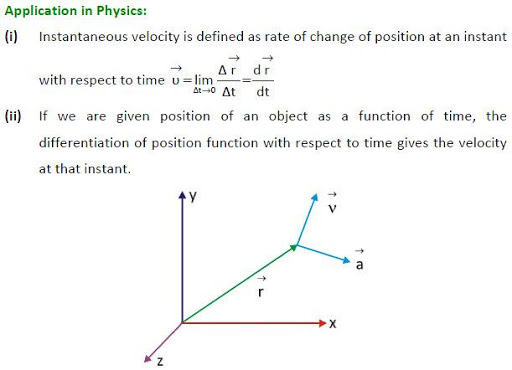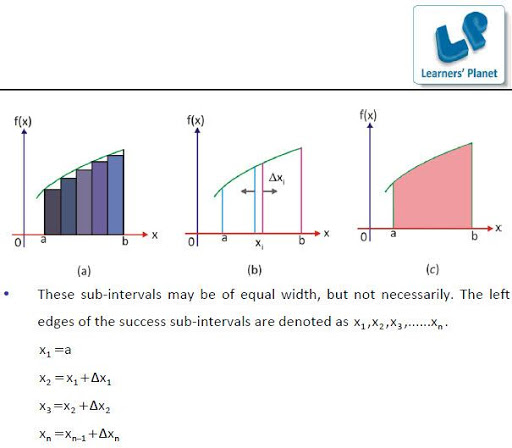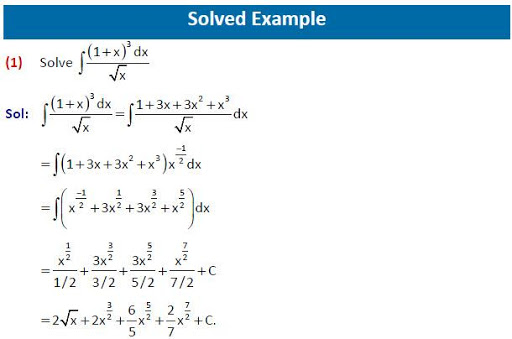### 免費玩JEE-MathematicalToolsinPhysics APP玩免費

#### 免費玩JEE-MathematicalToolsinPhysics App

Google Play
1.0
App下載
INR\$75.0
1970-01-012015-04-20